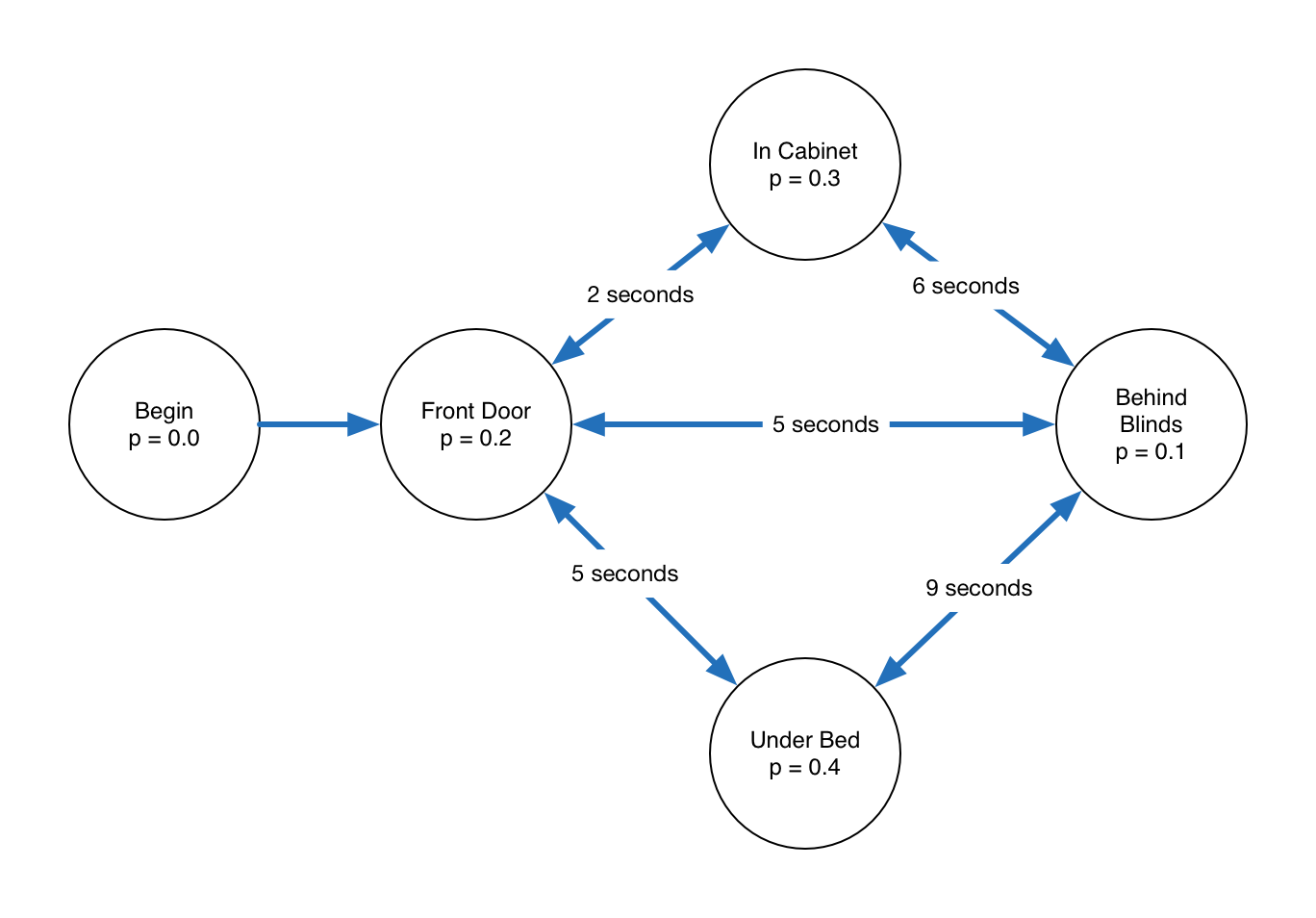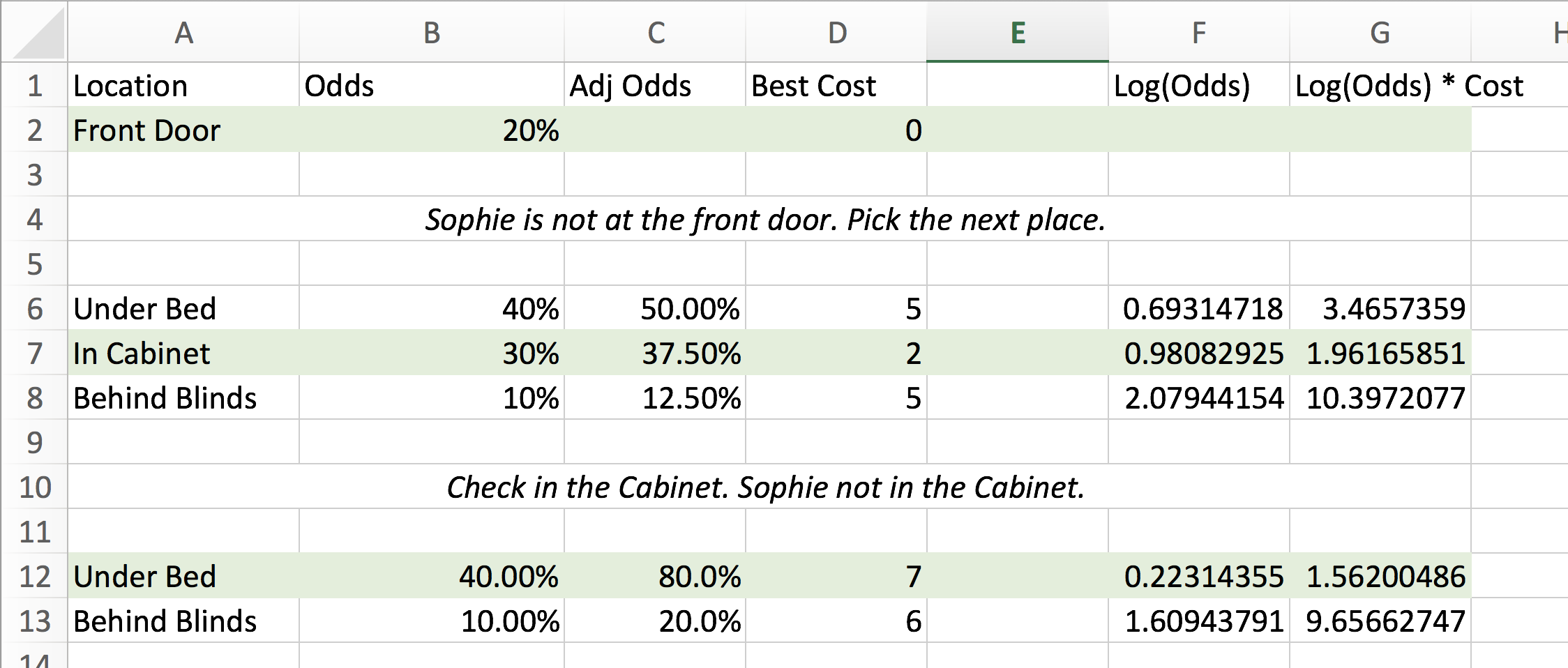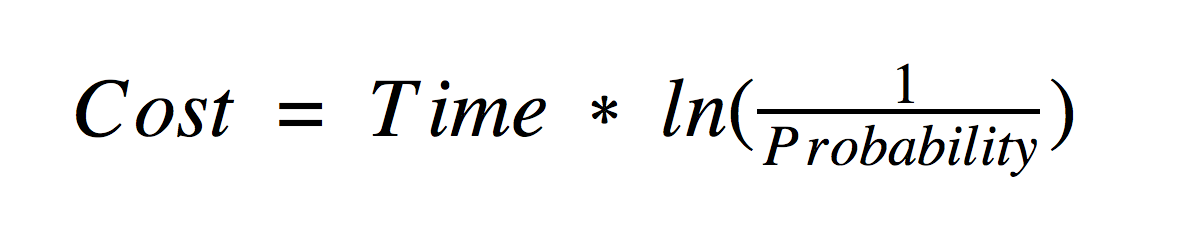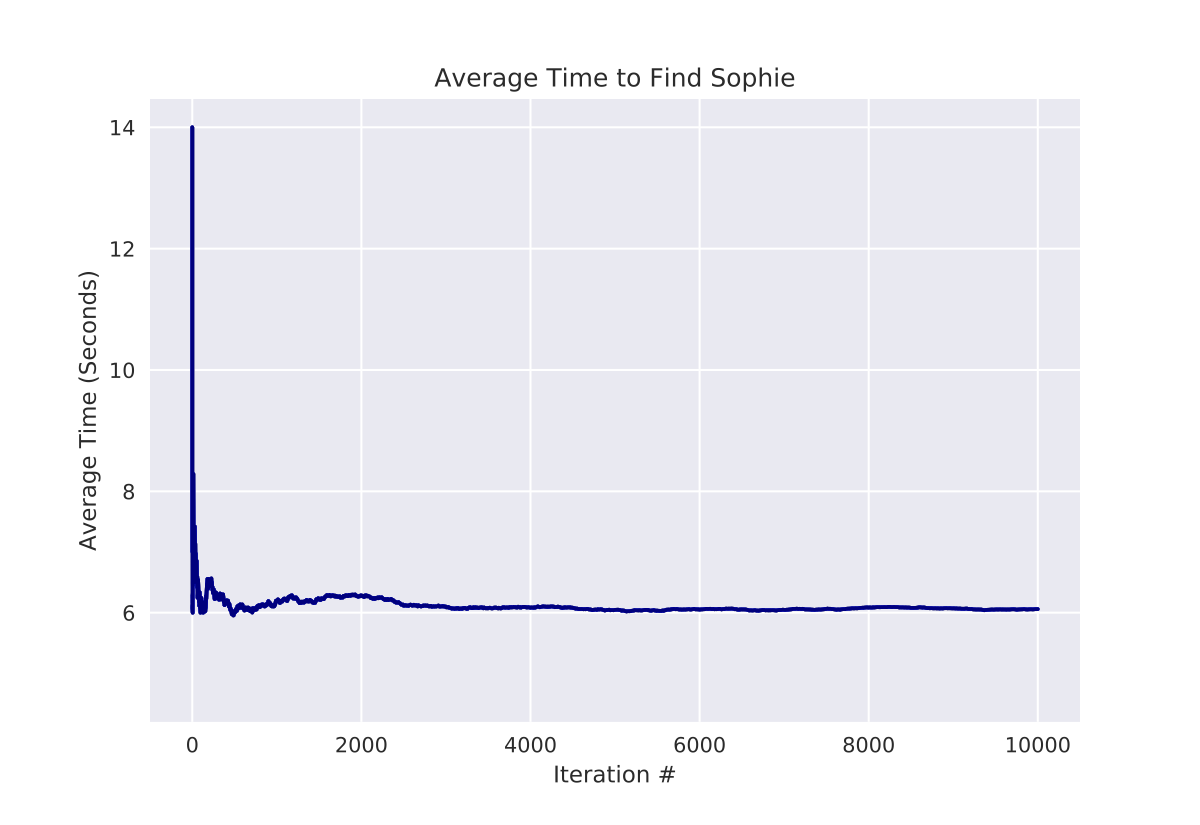# Traversing Graphs and Finding Cats

In its early days, Facebook listed some really interesting programming puzzles as part of their jobs site. One of the most interesting challenges I found was "Finding Sophie," a problem about finding the minimum expected cost to find an item randomly placed item in a graph - applied to finding where in your apartment your cat is hiding

I tried solving the problem when I first saw it in 2010, but I couldn't wrap my head around it. It has been on my "someday" list since then up until yesterday, when I finally solved it.

In this post, I'll go over modeling and solving the problem, as well as some possible areas for improvement.

### Overview

Summary of the problem:

Sophie (your cat) is a creature of habit. You know where all of her hiding places are, as well as the probability of her hiding in each one. You also know how long it takes you to walk from hiding place to hiding place. Write a program to determine the minimum expected time it will take to find Sophie in your apartment.

The full problem description is here: Finding Sophie - Facebook Engineering Challenges.

### Modeling

I decided to model this problem as an undirected graph since the costs are symmetric: the problem states that the time it takes to go from point A to point B is the same as the time to go from point B to point A. We're also given the probability that Sophie is in a given room.After modeling this as a graph, my first step was to try and solve the problem by hand. I wanted to see what algorithm I could come up with, and then once I was confident with it, implement it as code.

Here's the process I used to solve the problem manually:### Visiting a Room (or a node):

1. Check if Sophie is in that room.
2. Is Sophie in the room?
• Yes: Return the total time it has taken up to this point.
• No: Find the next “best” room to visit and travel there.

### Traveling to Another Room

Once we know where we want to go, find the shortest path to get there using Dijkstra's algorithm. Traverse this path, keeping in mind that we might visit other rooms along the way, and we may or may not have seen those rooms before.

### “Goodness” Heuristic

At this point, we have a basic algorithm, but we need some number that tells us the utility of a given path so that the algorithm can decide where to go next, taking into account travel time (in seconds), and the various probabilities that Sophie is there, keeping in mind that the probabilities change as we check rooms.

For example, if there are 3 rooms and the probabilities are uniformly distributed, there’s a ⅓ chance Sophie is in each room. Once we check a room and she is not there, there’s now a ½ chance Sophie is in each remaining room.

We now know 1) the probability that Sophie is in that room, and 2) the cost of getting there. In some ways, we want to pick the most likely place Sophie might be hiding, but at the same time, being efficient and not traveling needlessly. In order to combine those two heuristics, we can model them together as part of one cost heuristic:We can now model this as a minimization problem: when we’re picking between various alternatives and pick the one with the lowest cost. We're taking the inverse of the probability that Sophie is there. We do this because we want to maximize the probability, which is the same as minimizing the inverse of the probability. Next, we take the logarithm of this inverted probability so that the probabilities become additive.

Armed with our way-finding algorithm, we can now start implementing the solution:

# Solving the problem

I've uploaded my solution to Github. It's not "production-grade" code: it's far from tidy and is pretty inefficient at points, but done is better than perfect (for this problem, at least). With that warning out of the way, let's walk through the solution.

Parser code

The problem specifies how we will receive the input data:

We load this file into two data structures: rooms, which is a dictionary mapping the room to the initial probability Sophie is there, and arcs, which is a dictionary mapping a tuple (origin, destination) with travel time.

### Part 2: Basic Way-finding

Way-finding code

There are already some great Python packages available for way-finding, but since this was an intellectual vendetta, I decided to maximize satisfaction and write my own. To find the shortest path, we can implement Breadth-First Search (BFS) and use the travel time as the cost.

Once we have the way-finding, we can run a simulation:

### Part 3: Running One Iteration

Simulation code

Armed with the main pieces we need, we can run a simulation. Here's the path when Sophie is behind the blinds:

Under the bed:

### Part 4: Running Many Iterations and Finding the Average Time

Results as iPython Notebook

We’ve now done enough to find Sophie in any room of the house, and also return the total time taken to traverse the path. The problem asks us to find the average time, so we can run the experiment many times over and look at the average time.

Here's what the average travel time looks like over 10,000 iterations:We converge on 6.06 seconds based on this topology.

Another interesting discovery is that the paths are deterministic, meaning the algorithm will always take the same path. This intuitively makes sense since the only variable is where Sophie is hiding.

## Next Steps & Closing Thoughts

I think this mostly solves the problem. There might be a couple tweaks that we can do:

1. The solution is not particularly efficient. This works nicely with four nodes, but the complexity will blow up as we add more nodes. So there is a lot we can do to make the algorithm more efficient.
2. I think there are some tweaks that might make the cost heuristic more effective.
3. Most importantly, I wonder if there is a closed-form soution for this. We reached our answer through brute-force simulation, but I imagine there's a way you could find the answer as a closed-form equation and not have to run thousands of iterations of simulation.

Finally, a message to Facebook HR: Thanks for reading this! I’d be happy to accept an option grant backdated to 2009 when this problem was first posted :).